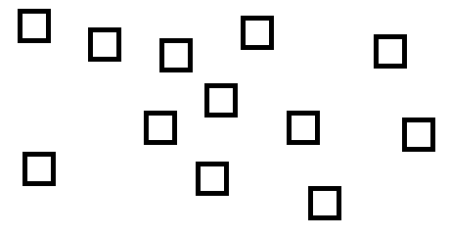Home > Grade 2 > Geometry > Constructing Rectangles

# Constructing Rectangles

Directions: Using the following squares, how many different rectangles can you make?### Hint

What are the properties of a rectangle?

### Answer

Answers will vary depending on uniqueness. Answers based on uniqueness are: 1×12, 2×6, 3×4, 4×3, 6×2, and 12×1. How does adding 4 more squares change the problem?

Source: Bryan Anderson

## Sum to 1,000 – Two Addends

Directions: Arrange the digits 1-6 into two 3-digit whole numbers. Make the sum as close …